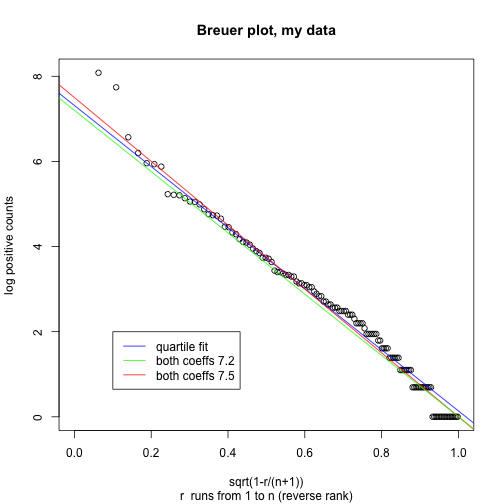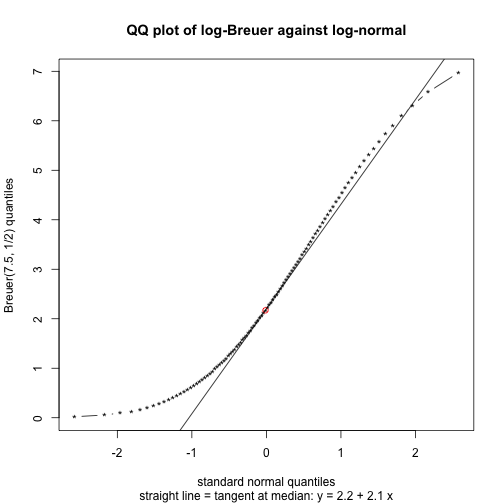### breuer.R

richard — Jan 9, 2014, 8:41 AM

``````## This script: http://rpubs.com/gill1109/11774

## Reference: "Empirical Patterns in Google Scholar Citation Counts"
## Peter T. Breuer and Jonathan P. Bowen
## Preprint available from Peter Breuer (University of Birmingham)

## Breuer plot: log positive citation count against square root of reverse rank number
## (most cited has reverse rank 0, next most cited 1, ...)
## Sometimes need a power different from 1/2

## My data (extracted from Google Scholar)
## http://www.math.leidenuniv.nl/~gill/citations.txt

## Previous analyses: http://rpubs.com/gill1109/11755

## The Breuer plot corresponds to the following model.
## Suppose U ~ Unif(0,1). Define X = c(1 - U^a), in my case, c = 7.5, a = 1/2.
## Then my positive citation counts are distributed as a sample of size N = 127
## from the distribution of exp(X), rounded up to positive whole numbers.

## After fitting this straight line to my data I simulate 9 times from the estimated model
## and show 9 Breuer plots (which of course give beautiful straight lines)
## and 9 Normal probability plots (which show a good fit to the log normal).

sep = "\$",
stringsAsFactors = FALSE,
blank.lines.skip = FALSE)
V1 <- data\$V1
counts <- as.numeric(V1[seq(from = 4, by = 7, to = 1100)])
counts[is.na(counts)] <- 0

## log positive citation counts, decreasing order

lpcounts <- sort(log(counts[counts>0]), decreasing = TRUE)

## create Breuer plot

plot(sqrt(ppoints(lpcounts)), lpcounts,
main = "Breuer plot, my data",
xlab = "sqrt(1-r/(n+1))",
ylab = "log positive counts",
sub = "r  runs from 1 to n (reverse rank)",
xlim = c(0, 1))

## Fit a straight line to body of data - determined by first, third quartile

## I'd like the straight line to go through the point (1, 0)
## So if it's y = a + bx I want a = -b
## Adjusting the quartiles line a bit to get equality I settle on a = 7.5

quartiles <- quantile(lpcounts, probs = c(0.75, 0.25))
quartiles
``````
``````  75%   25%
3.726 1.099
``````
``````
Uquartiles <- sqrt(c(0.25,0.75))
Uquartiles
``````
`````` 0.500 0.866
``````
``````
coefs <- lm(quartiles ~ Uquartiles)\$coef
coefs
``````
``````(Intercept)  Uquartiles
7.314      -7.177
``````
``````
abline(coefs, col = "blue")
abline(7.5, -7.5, col = "red")
abline(7.2, -7.2, col = "green")

legend(0.1, 2,
c("quartile fit", "both coeffs 7.2", "both coeffs 7.5"),
col = c("blue", "green", "red"),
lty = 1)
````````````
qqnorm(lpcounts,
main = "Normal probability plot, my data (log positive counts)",
xlab = "standard normal quantiles",
ylab = "log positive counts")
qqline(lpcounts)
````````````
## Now simulate (9 times) from the estimated model according to the Breuer plot:
## log positive count = lpc = 7.5 ( 1 - sqrt U) where U ~ Unif(0,1)
## Equivalently: (1 - (log pos cnt)/7.5)^2 ~ Unif(0,1)
## However to add realism at low counts I'll transform the continuous lpc to discrete
## by the transformation discrete lpc = log(ceiling(exp(continuous lpc)))

set.seed(1234)
N <- length(lpcounts)

par(mfrow = c(3, 3))
for (i in 1: 9) {
sim <- log(ceiling(exp(7.5 * (1 - sqrt(sort(runif(N)))))))
plot(sqrt(ppoints(sim)), sim,
main = "Breuer plot, simulated data",
xlab = "sqrt(1-r/(n+1))",
ylab = "log positive counts",
sub = "r  runs from 1 to n (reverse rank)",
xlim = c(0, 1))
abline(7.5, -7.5)
}
````````````
par(mfrow = c(3, 3))
set.seed(1234)
for (i in 1: 9) {
sim <- log(ceiling(exp(7.5 * (1 - sqrt(sort(runif(N)))))))
qqnorm(sim)
qqline(sim)
}
````````````
par(mfrow = c(1, 1))

## We noticed that the mean and standard devation of the normal seemed about equal.
## Is this a coincidence?
## Below I plot the QQ plot of the Breuer distribution (y-axis)
## against the standard normal (x-axis).
## The straight line is the tangent line at the medians.
## It is approximately y = 2.2 + 2.1 x
## So with this normal approximation, mu = 2.2 and sigma = 2.1
## Changing the power "1/2" a little, I could get them equal
## but changing the upper bound "7.5" does not help.

qBreuer <- 7.5 * (1 - sqrt(1-ppoints(100)))

qNorm <- qnorm(ppoints(100))

plot(qNorm, qBreuer, type="b", pch="*",
main = "QQ plot of log-Breuer against log-normal",
xlab = "standard normal quantiles",
ylab = "Breuer(7.5, 1/2) quantiles",
sub = "straight line = tangent at median: y = 2.2 + 2.1 x")

points(qNorm, qBreuer, col="red")

a <- 7.5*(1-sqrt(1/2))
b <- 7.5/(2*sqrt(pi))

a
``````
`````` 2.197
``````
``````b
``````
`````` 2.116
``````
``````abline(a, b)
``````